Thinking of Selling? Contact us today to explore our multiple options available!

# SEPT 14TH, ANTIQUES & COLLECTIBLES

### Auction Details

SIGNS, TOYS, ADVERTISING, OIL, GAS, ETC...SOMETHING FOR EVERYONE.
Choose Your Layout >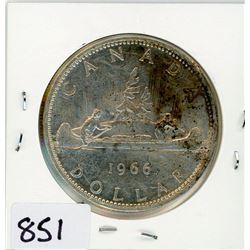851 - ONE DOLLAR COIN (CANADA) *1966* (SILVER)
Schmalz Auctions
ONE DOLLAR COIN (CANADA) *1966* (SILVER)
Bidding Has Concluded

Sold to Gstub for (10.00 + 1.30) x 1 = 11.30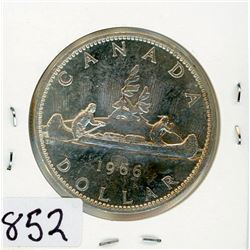852 - ONE DOLLAR COIN (CANADA) *1966* (SILVER)
Schmalz Auctions
ONE DOLLAR COIN (CANADA) *1966* (SILVER)
Bidding Has Concluded

Sold to Twigg for (12.50 + 1.63) x 1 = 14.13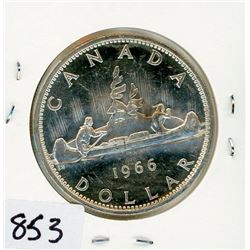853 - ONE DOLLAR COIN (CANADA) *1966* (SILVER)
Schmalz Auctions
ONE DOLLAR COIN (CANADA) *1966* (SILVER)
Bidding Has Concluded

Sold to Twigg for (17.50 + 2.28) x 1 = 19.78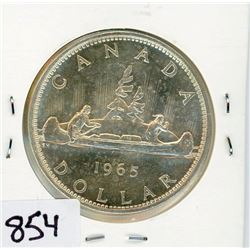854 - ONE DOLLAR COIN (CANADA) *1965* (SILVER)
Schmalz Auctions
ONE DOLLAR COIN (CANADA) *1965* (SILVER)
Bidding Has Concluded

Sold to Gstub for (10.00 + 1.30) x 1 = 11.30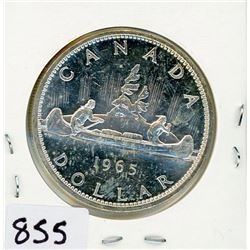855 - ONE DOLLAR COIN (CANADA) *1965* (SILVER)
Schmalz Auctions
ONE DOLLAR COIN (CANADA) *1965* (SILVER)
Bidding Has Concluded

Sold to Gstub for (10.00 + 1.30) x 1 = 11.30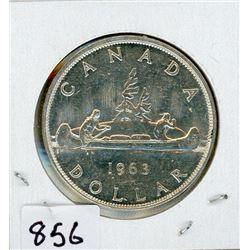856 - ONE DOLLAR COIN (CANADA) *1963* (SILVER)
Schmalz Auctions
ONE DOLLAR COIN (CANADA) *1963* (SILVER)
Bidding Has Concluded

Sold to floor for (12.50) x 1 = 12.50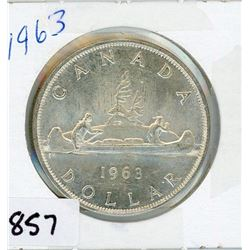857 - ONE DOLLAR COIN (CANADA) *1963* (SILVER)
Schmalz Auctions
ONE DOLLAR COIN (CANADA) *1963* (SILVER)
Bidding Has Concluded

Sold to floor for (12.50) x 1 = 12.50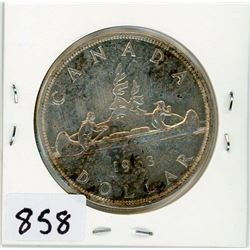858 - ONE DOLLAR COIN (CANADA) *1963* (SILVER)
Schmalz Auctions
ONE DOLLAR COIN (CANADA) *1963* (SILVER)
Bidding Has Concluded

Sold to floor for (12.50) x 1 = 12.50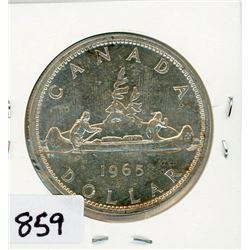859 - ONE DOLLAR COIN (CANADA) *1965* (SILVER)
Schmalz Auctions
ONE DOLLAR COIN (CANADA) *1965* (SILVER)
Bidding Has Concluded

Sold to floor for (12.50) x 1 = 12.50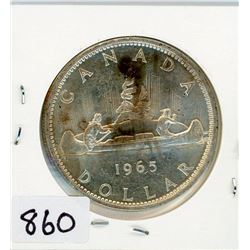860 - ONE DOLLAR COIN (CANADA) *1965* (SILVER)
Schmalz Auctions
ONE DOLLAR COIN (CANADA) *1965* (SILVER)
Bidding Has Concluded

Sold to Argillite for (12.50 + 1.63) x 1 = 14.13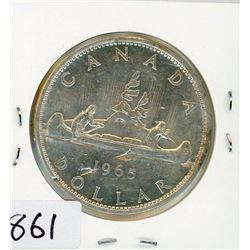861 - ONE DOLLAR COIN (CANADA) *1965* (SILVER)
Schmalz Auctions
ONE DOLLAR COIN (CANADA) *1965* (SILVER)
Bidding Has Concluded

Sold to floor for (12.50) x 1 = 12.50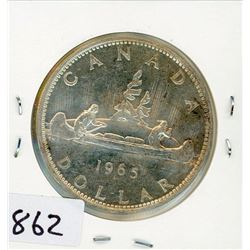862 - ONE DOLLAR COIN (CANADA) *1965* (SILVER)
Schmalz Auctions
ONE DOLLAR COIN (CANADA) *1965* (SILVER)
Bidding Has Concluded

Sold to Lindenguy for (12.50 + 1.63) x 1 = 14.13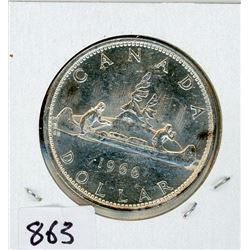863 - ONE DOLLAR COIN (CANADA) *1966* (SILVER)
Schmalz Auctions
ONE DOLLAR COIN (CANADA) *1966* (SILVER)
Bidding Has Concluded

Sold to floor for (12.50) x 1 = 12.50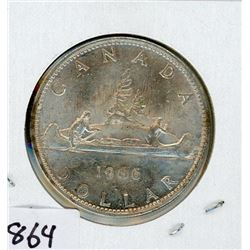864 - ONE DOLLAR COIN (CANADA) *1966* (SILVER)
Schmalz Auctions
ONE DOLLAR COIN (CANADA) *1966* (SILVER)
Bidding Has Concluded

Sold to floor for (12.50) x 1 = 12.50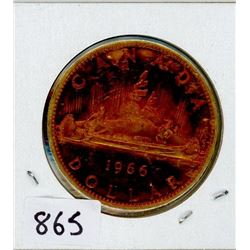865 - ONE DOLLAR COIN (CANADA) *1966* (SILVER)
Schmalz Auctions
ONE DOLLAR COIN (CANADA) *1966* (SILVER)
Bidding Has Concluded

Sold to Gstub for (10.00 + 1.30) x 1 = 11.30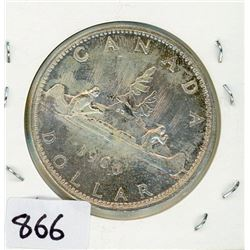866 - ONE DOLLAR COIN (CANADA) *1966* (SILVER)
Schmalz Auctions
ONE DOLLAR COIN (CANADA) *1966* (SILVER)
Bidding Has Concluded

Sold to floor for (12.50) x 1 = 12.50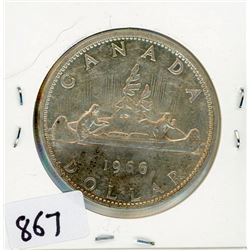867 - ONE DOLLAR COIN (CANADA) *1966* (SILVER)
Schmalz Auctions
ONE DOLLAR COIN (CANADA) *1966* (SILVER)
Bidding Has Concluded

Sold to floor for (12.50) x 1 = 12.50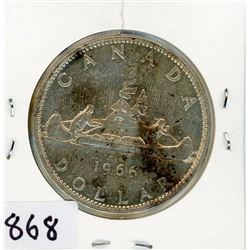868 - ONE DOLLAR COIN (CANADA) *1966* (SILVER)
Schmalz Auctions
ONE DOLLAR COIN (CANADA) *1966* (SILVER)
Bidding Has Concluded

Sold to floor for (12.50) x 1 = 12.50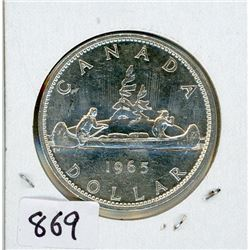869 - ONE DOLLAR COIN (CANADA) *1965* (SILVER)
Schmalz Auctions
ONE DOLLAR COIN (CANADA) *1965* (SILVER)
Bidding Has Concluded

Sold to Argillite for (20.00 + 2.60) x 1 = 22.60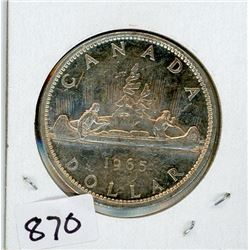870 - ONE DOLLAR COIN (CANADA) *1965* (SILVER)
Schmalz Auctions
ONE DOLLAR COIN (CANADA) *1965* (SILVER)
Bidding Has Concluded

Sold to floor for (12.50) x 1 = 12.50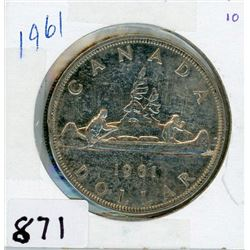871 - ONE DOLLAR COIN (CANADA) *1961* (SILVER)
Schmalz Auctions
ONE DOLLAR COIN (CANADA) *1961* (SILVER)
Bidding Has Concluded

Sold to floor for (12.50) x 1 = 12.50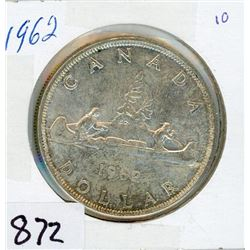872 - ONE DOLLAR COIN (CANADA) *1962* (SILVER)
Schmalz Auctions
ONE DOLLAR COIN (CANADA) *1962* (SILVER)
Bidding Has Concluded

Sold to floor for (12.50) x 1 = 12.50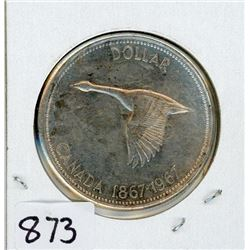873 - ONE DOLLAR COIN (CANADA) *1967* (SILVER)
Schmalz Auctions
ONE DOLLAR COIN (CANADA) *1967* (SILVER)
Bidding Has Concluded

Sold to floor for (12.50) x 1 = 12.50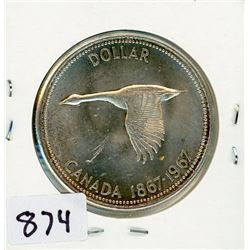874 - ONE DOLLAR COIN (CANADA) *1967* (SILVER)
Schmalz Auctions
ONE DOLLAR COIN (CANADA) *1967* (SILVER)
Bidding Has Concluded

Sold to floor for (12.50) x 1 = 12.50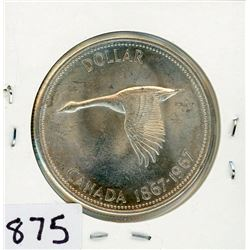875 - ONE DOLLAR COIN (CANADA) *1967* (SILVER)
Schmalz Auctions
ONE DOLLAR COIN (CANADA) *1967* (SILVER)
Bidding Has Concluded

Sold to floor for (12.50) x 1 = 12.50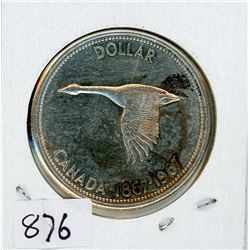876 - ONE DOLLAR COIN (CANADA) *1967* (SILVER)
Schmalz Auctions
ONE DOLLAR COIN (CANADA) *1967* (SILVER)
Bidding Has Concluded

Sold to Gstub for (10.00 + 1.30) x 1 = 11.30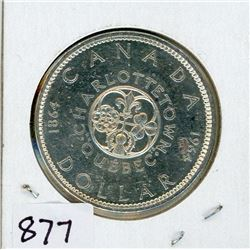877 - ONE DOLLAR COIN (CANADA) *1964* (SILVER)
Schmalz Auctions
ONE DOLLAR COIN (CANADA) *1964* (SILVER)
Bidding Has Concluded

Sold to Gstub for (10.00 + 1.30) x 1 = 11.30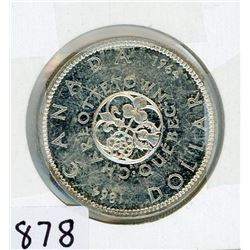878 - ONE DOLLAR COIN (CANADA) *1964* (SILVER)
Schmalz Auctions
ONE DOLLAR COIN (CANADA) *1964* (SILVER)
Bidding Has Concluded

Sold to Gstub for (10.00 + 1.30) x 1 = 11.30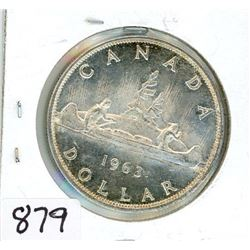879 - ONE DOLLAR COIN (CANADA) *1963* (SILVER)
Schmalz Auctions
ONE DOLLAR COIN (CANADA) *1963* (SILVER)
Bidding Has Concluded

Sold to Gstub for (10.00 + 1.30) x 1 = 11.30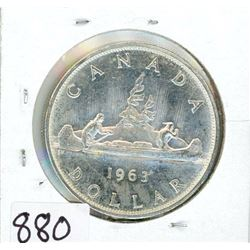880 - ONE DOLLAR COIN (CANADA) *1963* (SILVER)
Schmalz Auctions
ONE DOLLAR COIN (CANADA) *1963* (SILVER)
Bidding Has Concluded

Sold to floor for (12.50) x 1 = 12.50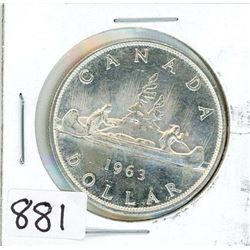881 - ONE DOLLAR COIN (CANADA) *1963* (SILVER)
Schmalz Auctions
ONE DOLLAR COIN (CANADA) *1963* (SILVER)
Bidding Has Concluded

Sold to floor for (12.50) x 1 = 12.50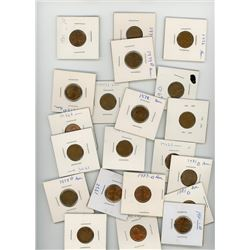882 - LOT OF 40 AMERICAN PENNIES ( (VARIOUS DATES BETWEEN 1964-1983)
Schmalz Auctions
LOT OF 40 AMERICAN PENNIES ( (VARIOUS DATES BETWEEN 1964-1983)
Bidding Has Concluded

Sold to floor for (5.00) x 1 = 5.00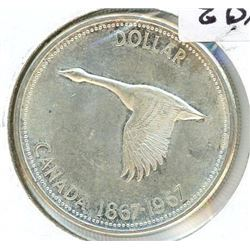883 - ONE DOLLAR COIN (CANADA) *1967* (SILVER)
Schmalz Auctions
ONE DOLLAR COIN (CANADA) *1967* (SILVER)
Bidding Has Concluded

Sold to floor for (12.50) x 1 = 12.50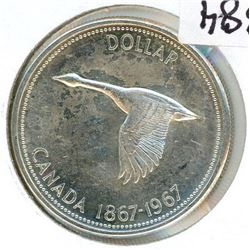884 - ONE DOLLAR COIN (CANADA) *1967* (SILVER)
Schmalz Auctions
ONE DOLLAR COIN (CANADA) *1967* (SILVER)
Bidding Has Concluded

Sold to morris0YF for (12.50 + 1.63) x 1 = 14.13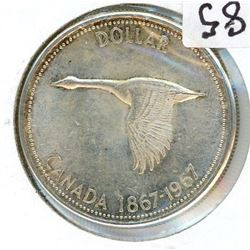885 - ONE DOLLAR COIN (CANADA) *1967* (SILVER)
Schmalz Auctions
ONE DOLLAR COIN (CANADA) *1967* (SILVER)
Bidding Has Concluded

Sold to floor for (12.50) x 1 = 12.50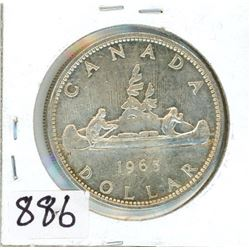886 - ONE DOLLAR COIN (CANADA) *1965* (SILVER)
Schmalz Auctions
ONE DOLLAR COIN (CANADA) *1965* (SILVER)
Bidding Has Concluded

Sold to SuperDaveMc for (12.50 + 1.63) x 1 = 14.13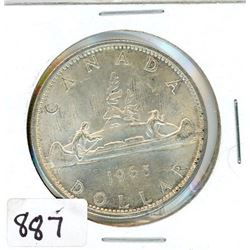887 - ONE DOLLAR COIN (CANADA) *1965* (SILVER)
Schmalz Auctions
ONE DOLLAR COIN (CANADA) *1965* (SILVER)
Bidding Has Concluded

Sold to SuperDaveMc for (12.50 + 1.63) x 1 = 14.13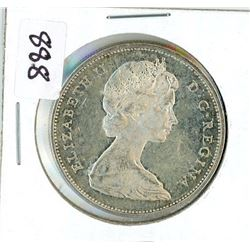888 - ONE DOLLAR COIN (CANADA) *1965* (SILVER)
Schmalz Auctions
ONE DOLLAR COIN (CANADA) *1965* (SILVER)
Bidding Has Concluded

Sold to SuperDaveMc for (12.50 + 1.63) x 1 = 14.13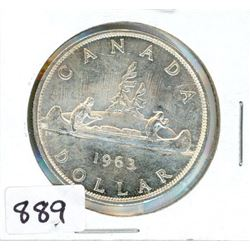889 - ONE DOLLAR COIN (CANADA) *1963* (SILVER)
Schmalz Auctions
ONE DOLLAR COIN (CANADA) *1963* (SILVER)
Bidding Has Concluded

Sold to SuperDaveMc for (12.50 + 1.63) x 1 = 14.13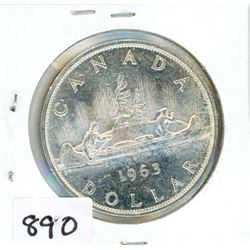890 - ONE DOLLAR COIN (CANADA) *1963* (SILVER)
Schmalz Auctions
ONE DOLLAR COIN (CANADA) *1963* (SILVER)
Bidding Has Concluded

Sold to SuperDaveMc for (15.00 + 1.95) x 1 = 16.95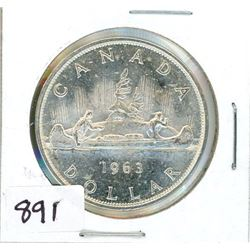891 - ONE DOLLAR COIN (CANADA) *1963* (SILVER)
Schmalz Auctions
ONE DOLLAR COIN (CANADA) *1963* (SILVER)
Bidding Has Concluded

Sold to SuperDaveMc for (15.00 + 1.95) x 1 = 16.95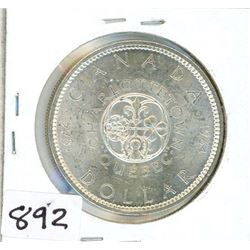892 - ONE DOLLAR COIN (CANADA) *1964* (SILVER)
Schmalz Auctions
ONE DOLLAR COIN (CANADA) *1964* (SILVER)
Bidding Has Concluded

Sold to morris0YF for (14.00 + 1.82) x 1 = 15.82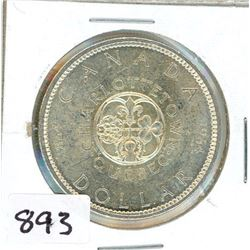893 - ONE DOLLAR COIN (CANADA) *1964* (SILVER)
Schmalz Auctions
ONE DOLLAR COIN (CANADA) *1964* (SILVER)
Bidding Has Concluded

Sold to SuperDaveMc for (14.00 + 1.82) x 1 = 15.82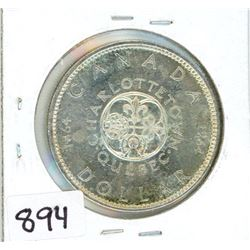894 - ONE DOLLAR COIN (CANADA) *1964* (SILVER)
Schmalz Auctions
ONE DOLLAR COIN (CANADA) *1964* (SILVER)
Bidding Has Concluded

Sold to SuperDaveMc for (15.00 + 1.95) x 1 = 16.95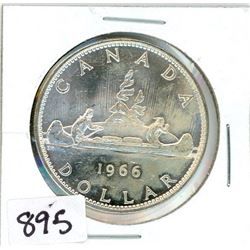895 - ONE DOLLAR COIN (CANADA) *1966* (SILVER)
Schmalz Auctions
ONE DOLLAR COIN (CANADA) *1966* (SILVER)
Bidding Has Concluded

Sold to SuperDaveMc for (12.50 + 1.63) x 1 = 14.13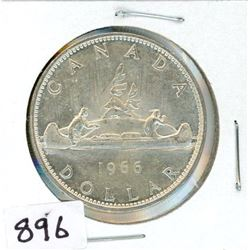896 - ONE DOLLAR COIN (CANADA) *1966* (SILVER)
Schmalz Auctions
ONE DOLLAR COIN (CANADA) *1966* (SILVER)
Bidding Has Concluded

Sold to SuperDaveMc for (12.50 + 1.63) x 1 = 14.13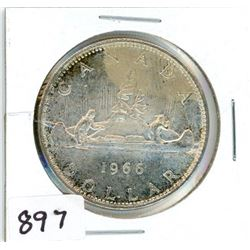897 - ONE DOLLAR COIN (CANADA) *1966* (SILVER)
Schmalz Auctions
ONE DOLLAR COIN (CANADA) *1966* (SILVER)
Bidding Has Concluded

Sold to floor for (12.50) x 1 = 12.50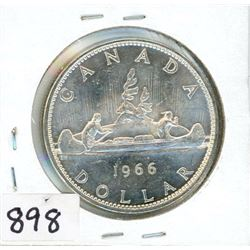898 - ONE DOLLAR COIN (CANADA) *1966* (SILVER)
Schmalz Auctions
ONE DOLLAR COIN (CANADA) *1966* (SILVER)
Bidding Has Concluded

Sold to SuperDaveMc for (12.50 + 1.63) x 1 = 14.13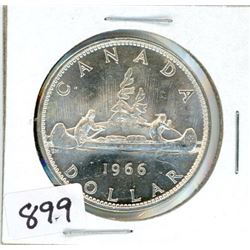899 - ONE DOLLAR COIN (CANADA) *1966* (SILVER)
Schmalz Auctions
ONE DOLLAR COIN (CANADA) *1966* (SILVER)
Bidding Has Concluded

Sold to floor for (15.00) x 1 = 15.00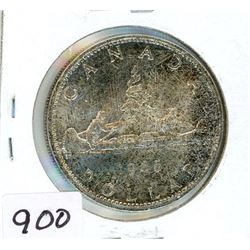900 - ONE DOLLAR COIN (CANADA) *1966* (SILVER)
Schmalz Auctions
ONE DOLLAR COIN (CANADA) *1966* (SILVER)
Bidding Has Concluded

Sold to SuperDaveMc for (12.50 + 1.63) x 1 = 14.13